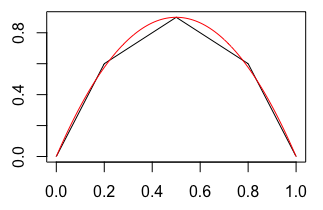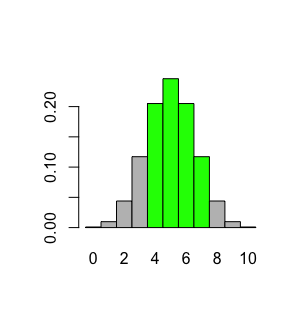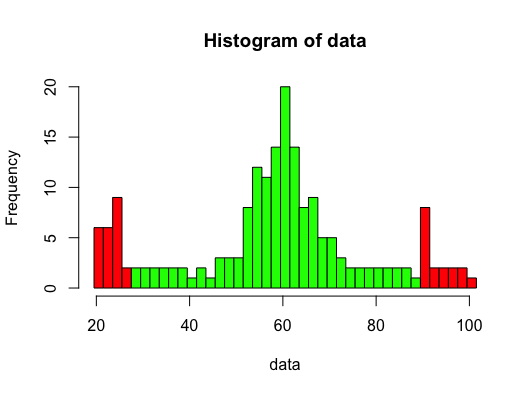Exercises - Functions and Conditionals

1. Predict the output of the following when executed in R:

different.craps = function(roll) {
if (any(c(8,9) == roll))
return("win")
if (any(c(2,11,12) == roll))
return("lose")
else
return("keep rolling")
}

different.craps(7)

"keep rolling"

2. Write a function U(n) that calculates the following:

$$U(n) = \left\{ \begin{array}{cl} 7n+3 & \quad \textrm{if n is a multiple of 3}\\\\ \displaystyle{\frac{7n+2}{3}} & \quad \textrm{if n has remainder 1 upon division by 3}\\\\ \displaystyle{\frac{n-2}{3}} & \quad \textrm{if n has remainder 2 upon division by 3} \end{array} \right.$$
U = function(n) {
remainder = n %% 3
if (remainder == 0) {
return(7*n+3)
} else if (remainder == 1) {
return((7*n+2)/3)
} else {
return((n-2)/3)
}
}

3. A coin is flipped and one of the results "heads", "tails", "on edge" is stored in the variable coin. Write code that prints "It will be sunny" if the coin landed "heads", prints "It will be cloudy" if the coin landed "tails", and prints "There will be a blizzard" if the coin landed "on edge".

print("It will be sunny")
} else if (coin=="tails") {
print("It will be cloudy")
} else {
print("There will be a blizzard")
}

4. Sometimes one can find it useful to use a piecewise-linear function to approximate some other function.Above we see the function shown in red, and given by $$f(x) = 0.9 - \frac{18}{5} (x- 0.5)^2$$ can be fairly well approximated by the piecewise-linear function shown in black, and given by $$f_{approx}(x) = \left\{ \begin{array}{cl} 3x & \textrm{ if } \quad x \lt 0.2\\ 0.4 + x & \textrm{ if } \quad 0.2 \le x \lt 0.5\\ 1.4 - x & \textrm{ if } \quad 0.5 \le x \lt 0.8\\ 3 - 3x & \textrm{ otherwise } \end{array} \right.$$ Write two functions f(x) and f.approx(x) that evaluate $f(x)$ and $f_{approx}(x)$ as defined above, respectively.

Upon doing this correctly, the following R code should produce the same plot as shown at the beginning of this problem:

xs = seq(from=0,to=1,by=0.01)
ys = sapply(xs,f.approx)
plot(xs,ys,type="l",xlab="",ylab="")
y2s = sapply(xs,f)
lines(xs,y2s,col="red")

One should be careful, however, about combining multiple applications of the approximating function -- the little errors that result from each application can add up.

To see this, use R to find the sum, to the nearest thousandth, of the differences $(f(x)-f_{approx}(x))$ as $x$ ranges over the values $0, 0.01, 0.02, 0.03, \ldots, 0.99, 1$.

As a check of your code, the same sum when $x$ ranges over the values $0, 0.1, 0.2, \ldots, 0.9, 1$ should be $0.24$.

f.approx = function(x) {
if (x < 0.2) {
return(3*x)
} else if ((0.2 <= x) && (x < 0.5)) {
return(0.4+x)
} else if ((0.5 <= x) && (x < 0.8)) {
return(1.4 - x)
} else {
return(3-3*x)
}
}

f = function(x) {
.9-(18/5)*(x-.5)^2
}

xs = seq(from=0,to=1,by=0.01)
sum(sapply(xs,f)-sapply(xs,f.approx))

5. Consider the data set of integers given here. In an effort to visualize where the outliers fall for a given data set, create a function outliers.of(data,lump.num) that produces a histogram of the elements in the vector named data in the following way:

1. The breaks used in the histogram should be constructed so that lump.num consecutive integers are "lumped together" in each rectangle of the histogram.

For example, if lump.num is 1 and the minimum value in the data set is 7, then the breaks between the rectangles should occur at $\{6.5, 7.5, 8.5, \ldots\}$, ending with max(data)+0.5. Thus, 7 is the center of the first rectangle, 8 the center of the second, 9 for the third, and so on.

If instead the lump.num is 2 and and the minimum is again 7, the breaks should occur at $\{6.5, 8.5, 10.5, \ldots\}$, again ending with max(data)+1.5. In this case, both 7 and 8 are in the first rectangle, while 9 and 10 are in the second, and so on.

Beyond getting lump.num consecutive integers associated with each rectangle, note that the "lumped together" $x$ values being counted will be centered in the rectangle to which they correspond.

Note that, as a bit of an exception, the last value should always be chosen to be lump.num-0.5 past the maximum value in the data set. This is so R doesn't complain that there are data values outside the range breaks specifies.

2. One can pass a col argument that consists of a vector of color names (as strings of text) to many functions that plot things (e.g., boxplot(), hist(), plot(), etc.). An example is shown below using the boxplot() function:

binom.region = function(a,b,n,p) {
xs = 0:n
probs = dbinom(xs,size=n,prob=p)
cols = ifelse((xs >= a) & (xs <= b),"green","gray")
barplot(names.arg=xs,height=probs,space=0,col=cols)
}

binom.region(4,7,10,0.5)

which results in the following plot:The col argument works similarly for the hist() function, coloring consecutive bars with the colors the col vector specifies.

Use this argument to color the bars "red" if any of the numbers to which they correspond would be outlier values by the IQR test, and "green" otherwise.

3. The function should return a vector of all outliers values (by the IQR test) present in the data set.

If this function is used on this example data set with lump.num=2, the result should look like this:outliers.of(example.data,lump.num=2)
  92  94  23  24  25  25  25  22  20  25  20  26  22  98
  22  25  25  97  22  93  20  20  95  25  20  20  22  96
  25  99 100

Note that above, there are 10 bars that are colored red and 31 outlier values found. When one applies the function to the data set provided at the beginning of this problem, also using a lump.num=2, what is the sum of the number of bars that are red and the number of outlier values found?

outliers.of = function(data,lump.num) {
lower.bound = quantile(data,0.25)-1.5*IQR(data)
upper.bound = quantile(data,0.75)+1.5*IQR(data)

iqr.outliers = data[data < lower.bound | data > upper.bound]

bar.bounds = seq(from=min(data)-0.5,to=max(data)+lump.num-0.5,by=lump.num)
right.bar.bounds = bar.bounds[-1]
left.bar.bounds = bar.bounds[-length(bar.bounds)]

cols = ifelse((left.bar.bounds > upper.bound |
right.bar.bounds < lower.bound),"red","green")

h = hist(data,breaks=bar.bounds,col=cols)
return(iqr.outliers)
}

data = ...

outliers.of(data,lump.num=2)
length(outliers.of(data,lump.num=2))

Under certain circumstances in R (and other programming languages) one can use a function being defined in the definition of that function. When a function is defined in this way, we say the definition is recursive. Allowing such definitions might initially sound absurd , but it is actually perfectly reasonable. Consider how one might define the factorial function $n!$ with the following:

$$n! = \left\{ \begin{array}{ll} 1 & \textrm{ if } n = 1\\ n \cdot (n-1)! & \textrm{ otherwise} \end{array} \right.$$

Note how $(n-1)!$ appears in the definition of $n!$. Using the above, we can find $4!$ by applying the above successively to $4!$, $3!$, $2!$, and finally $1!$, as shown below: $$\begin{array}{rcll} 4! &=& 4 \cdot 3!\\ &=& 4 \cdot (3 \cdot 2!)\\ &=& 4 \cdot (3 \cdot (2 \cdot 1!))\\ &=& 4 \cdot (3 \cdot (2 \cdot 1)))\\ &=& 4 \cdot 3 \cdot 2 \cdot 1\\ \end{array}$$ We could define the factorial function recursively in R in a similar way:
fact = function(n) {
ifelse(n==1,1,n*fact(n-1))
}

Applying this function to find $5!$, we have
fact(5)
 120

Be careful though -- what we have written above is not actually equivalent to factorial(n) in R, as it is not "vector-friendly". To see this, run both factorial(c(3,4)) and fact(c(3,4)). Can you figure out why you get what you get?

Here's another recursive definition. This time, the function reverses the order of the elements of a vector:

reverse = function(v) {
if (length(v) == 1) {
return(v)
} else {
return(c(v[length(v)],reverse(v[1:(length(v)-1)])))
}
}

reverse(1:10)
 10  9  8  7  6  5  4  3  2  1

To see how the above function works, consider the following sequences formed during its invocation:
reverse(c(1,2,3,4,5)) = c(5, reverse(c(1,2,3,4)))
= c(5, c(4, reverse(c(1,2,3))))
= c(5, c(4, c(3, reverse(c(1,2)))))
= c(5, c(4, c(3, c(2, reverse(c(1))))))
= c(5, c(4, c(3, c(2, 1))))
= c(5, c(4, c(3,2,1)))
= c(5, c(4,3,2,1))
= c(5,4,3,2,1)

As shown above, the key to writing a recursive function has two parts:

1. First, one has to deal with the smallest case that could happen. In the case of the factorial function, the smallest number we decided to worry about was $1$. In the case of reverse(), the smallest case was a list of only one element.

In both of these "smallest cases", the answer was immediate, $1! = 1$ and the reverse of a list of only one element is itself.

2. Second, after dealing with the smallest case, one defines the function for some input in terms of the same function applied to some "smaller" case.

In the case of the factorial function, we defined $f(n)$ in terms of $f(n-1)$, and clearly $(n-1)$ is smaller in magnitude than $n$ for the inputs our function considers.

In the case of reversing a list, we defined reverse(n) in terms of the same function applied to a vector with one less element (i.e., v[1:(length(v)-1)]).

With all of this in mind, suppose one is interested in automating the process of finding out how often certain sums occur when $n$ dice are rolled.

For example, if only $n=1$ die is rolled, then there are six equally likely possibilities: $$1,2,3,4,5,\textrm{ and } 6$$ If two dice are rolled, one often organizes all of the equally likely possibilities in a table: $$\begin{array}{c|c|c|c|c|c|c|} & 1 & 2 & 3 & 4 & 5 & 6\\\hline 1 & 2 & 3 & 4 & 5 & 6 & 7\\\hline 2 & 3 & 4 & 5 & 6 & 7 & 8\\\hline 3 & 4 & 5 & 6 & 7 & 8 & 9\\\hline 4 & 5 & 6 & 7 & 8 & 9 & 10\\\hline 5 & 6 & 7 & 8 & 9 & 10 & 11\\\hline 6 & 7 & 8 & 9 & 10 & 11 & 12\\\hline \end{array}$$ If more than two dice are rolled, however, organizing things into a table form becomes more difficult.

Imagine a function that would produce the same values, but in a sorted vector of equally likely rolls -- as the following suggests:

sums.of.n.dice(1)
 1 2 3 4 5 6

sums.of.n.dice(2)
  2  3  3  4  4  4  5  5  5  5  6  6  6  6  6  7  7  7  7  7  7  8
  8  8  8  8  9  9  9  9 10 10 10 11 11 12

sums.of.n.dice(3)
  3  4  4  4  5  5  5  5  5  5  6  6  6  6  6  6  6  6  6  6  7  7
  7  7  7  7  7  7  7  7  7  7  7  7  7  8  8  8  8  8  8  8  8  8
  8  8  8  8  8  8  8  8  8  8  8  8  9  9  9  9  9  9  9  9  9  9
  9  9  9  9  9  9  9  9  9  9  9  9  9  9  9 10 10 10 10 10 10 10
 10 10 10 10 10 10 10 10 10 10 10 10 10 10 10 10 10 10 10 10 11 11
 11 11 11 11 11 11 11 11 11 11 11 11 11 11 11 11 11 11 11 11 11 11
 11 11 11 12 12 12 12 12 12 12 12 12 12 12 12 12 12 12 12 12 12 12
 12 12 12 12 12 12 13 13 13 13 13 13 13 13 13 13 13 13 13 13 13 13
 13 13 13 13 13 14 14 14 14 14 14 14 14 14 14 14 14 14 14 14 15 15
 15 15 15 15 15 15 15 15 16 16 16 16 16 16 17 17 17 18

Use a recursive definition in R to define such a function and then use it to find out how many ways one can roll a 19 with 5 standard dice.

: One could make the argument that we should have started with $n=0$ as our smallest case, since $0!=1$. This is perfectly reasonable, and modifying the function to accomplish this is easily done. As a side note related to this, know that there is a way to define the factorial function for non-integers as well! Doing so involves something called the Gamma function, $\Gamma(x)$. Compare gamma(1:10) to factorial(1:10) in R to see the relationship between these two functions, and then evaluate gamma(sqrt(2)) to see the gamma function applied to a non-integer. If you are curious and wish to learn more, do an internet search for "gamma function", and see what you find!

sums.of.n.dice = function(n) {
if (n == 1) {
return(1:6)
} else {
return(sort(rep(sums.of.n.dice(n-1),each=6)+(1:6)))
}
}

rolls = sums.of.n.dice(5)
sum(rolls == 19)

7. A palindrome is a word or phrase that reads the same forwards or backwards, such as "madam" or "nurses run". Similarly, A palindromic number is a number that is the same when written forwards or backwards. The first few palindromic numbers are $$0, 1, 2, 3, 4, 5, 6, 7, 8, 9, 11, 22, 33, 44, 55, 66, 77, 88, 99, 101, 111, 121, \ldots$$ Suppose you are curious about the proportion of palindromic numbers from 1 to some given $n$.

You suspect the following function, that reverse the order of elements in a vector might be helpful:

reverse = function(v) {
if (length(v) == 1) {
return(v)
} else {
return(c(v[length(v)],reverse(v[1:(length(v)-1)])))
}
}

reverse(1:10)
 10  9  8  7  6  5  4  3  2  1

With this in mind, write the following two functions in R to aid you in deciding if a given value is a palindromic number:
1. digits.of(n), which returns a vector with the digits of $n$ as its elements. The following provides an example of its application:

digits.of(56789)
 5 6 7 8 9

2. is.palindromic.num(n), which returns either $TRUE$ or $FALSE$ depending on whether $n$ is a palindromic number or not, respectively, consistent with the below examples:

is.palindromic.num(122787221)
 TRUE

is.palindromic.num(122687221)
 FALSE

Use these two functions to answer the question "How many palindromic numbers are there from $1$ to $54321$?"

reverse = function(v) {
if (length(v) == 1) {
return(v)
} else {
return(c(v[length(v)],reverse(v[1:(length(v)-1)])))
}
}

digits.of = function(n) {
num.digits = ifelse(n==0,0,trunc(log(n,base=10))+1)
ks = num.digits:1
digits = (n %% (10^ks)) %/% (10^(ks-1))
return(digits)
}

is.palindromic.num = function(n) {
return(all(digits.of(n) == reverse(digits.of(n))))
}

sum(sapply(1:54321,is.palindromic.num))